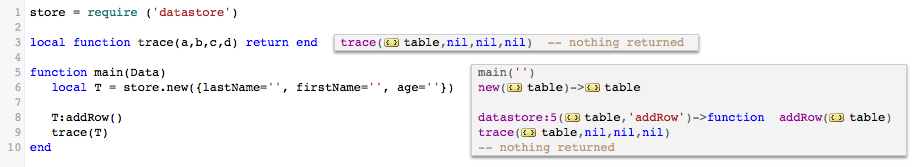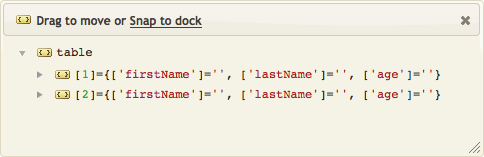# Trick for maintaining a Lua table with ‘rows’

We had a customer that was looking to maintain a 2 dimensional table. The built in Lua table is a good structure for this.

They wanted to know an easy way to add additional structured rows to this array. We put together this example for them using a ‘datastore’ module.

To make things work ‘under the hood’ in an elegant way we had to use a feature of Lua called ‘Meta Tables’. Meta tables allow a Lua programmer to simulate objects and classes in Lua. Let’s see the code in action:The `datastore.new()` function returns us a table array which has the first entry set to the ‘row’ table passed in. The table array has a meta table with the __index entry set up. The __index function is called when we access a property of the table which doesn’t exist. So in the case of “addRow” it returns the `addRow()` function. Calling `:addRow()` means this function is executed on the table itself. The function clones the first row by iterating through all it’s keys so we end up with another ’empty’ row. This the table produced by that code:Here is the source code for main:

```store = require ('datastore')

function main(Data)
local T = store.new({lastName='', firstName='', age=''})

trace(T)
end```

Here is the source code for the module:

```local datastore={}

local MT = {}

function MT.__index(T, K)
return MT[K]
end

T[#T+1] = {}
local R = T[#T]
for K,_ in pairs(T) do
R[K] = ''
end
end

function datastore.new(Row)
local T = {Row}
setmetatable(T, MT)
return T
end

return datastore```